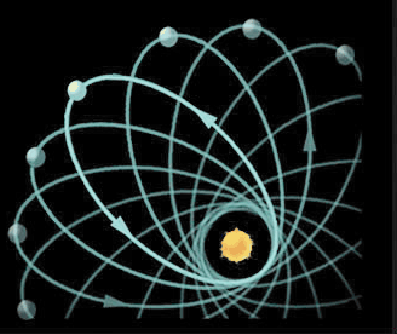## Precession of the perihelion of Mercury

A long-standing problem in the study of the Solar System was that the orbit of Mercury did not behave as required by Newton's equations.

To understand what the problem is let me describe the way Mercury's orbit looks. As it orbits the Sun, this planet follows an ellipse...but only approximately: it is found that the point of closest approach of Mercury to the sun does not always occur at the same place but that it slowly moves around the sun (see Fig. 7.20). This rotation of the orbit is called a precession.

The precession of the orbit is not peculiar to Mercury, all the planetary orbits precess. In fact, Newton's theory predicts these effects, as being produced by the pull of the planets on one another. The question is whether Newton's predictions agree with the amount an orbit precesses; it is not enough to understand qualitatively what is the origin of an effect, such arguments must be backed by hard numbers to give them credence. The precession of the orbits of all planets except for Mercury's can, in fact, be understood using Newton;s equations. But Mercury seemed to be an exception.As seen from Earth the precession of Mercury's orbit is measured to be 5600 seconds of arc per century (one second of arc=1/3600 degrees). Newton's equations, taking into account all the effects from the other planets (as well as a very slight deformation of the sun due to its rotation) and the fact that the Earth is not an inertial frame of reference, predicts a precession of 5557 seconds of arc per century. There is a discrepancy of 43 seconds of arc per century.

This discrepancy cannot be accounted for using Newton's formalism. Many ad-hoc fixes were devised (such as assuming there was a certain amount of dust between the Sun and Mercury) but none were consistent with other observations (for example, no evidence of dust was found when the region between Mercury and the Sun was carefully scrutinized). In contrast, Einstein was able to predict, without any adjustments whatsoever, that the orbit of Mercury should precess by an extra 43 seconds of arc per century should the General Theory of Relativity be correct.

An early success of Einstein's theory was an explanation for the 43'' per century precession of the perihelion of Mercury. In a curved spacetime a planet does not orbit the Sun in a static elliptical orbit, as in Newton's theory. Rather, the orbit is obliged to precess because of the curvature of spacetime. When Einstein calculated the magnitude of this effect for Mercury he got precisely the previously unexplained 43''. He correctly took the view that this was an important confirmation of his theory.

Einstein's theory also correctly accounts for a smaller discrepancy of 8.6'' per century in the precession of the perihelion of Venus. The value is smaller than that of Mercury because Venus is further from the Sun and the curvature of spacetime is less.